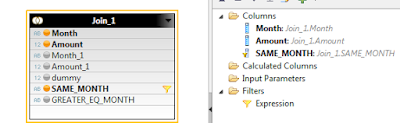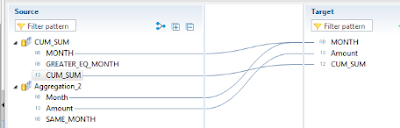Saturday, 9 July 2016

Cumulative Sum / Running Total in HANA Calculation View

Requirement :

Most of the popular reporting tools can handle cumulative sum or running total , but still there have been lot of posts in SCN about whether it is possible to do it in HANA itself.  So, just wanted to see , if it can be easily done via Graphical Calculation view and it seems to be working .

Note: This might still be easier to do in Scripted View or Reporting Tool .

In Figure 1, our base data is in Column 1 and 2 and we want to add Colum 3 ( "C UM_SUM" ) to existing data set.Figure 1 : Base data and result

Detail Steps :
Figure 2 shows overall design of the calculation view.  It is pretty simple one, isn't it ?Figure 2 : Graphical Calculation View
a) I have two projections (  ALL_DATA and ALL_DATA2 )  of the base data ( first two columns in Figure 1 )
b) Created one Calculated Column "DUMMY" on each projection and assigned it a value 1 .  You can assign any other constant value .
c) In join node ( JOIN_1 ) ,(inner)  joined these two data sets via 'DUMMY" column  .  After joining output would be like below

If you notice, for every month now I shall have 12 records  ( overall 12 X 12 = 144 ) .  Where my Month matches with Month1 , that is my actual value for that month . And I need to sum all other values where  Month >= Month1 .  So, for above example, sum of  10, 20 and 30 would give me cumulative sum for Month 3.
To do this, I defined two calculated attributes  , one to check if Month = Month1  and another to check If Month >= Month1  ( refer Figure 4 ) .Figure 4: Calculated Columns ( Attributes) on Join_1 node
d) Now, I have two aggregation nodes. One with filter SAME_MONTH = "Y' and another with GREATER_EQ_MONTH = 'Y' .Figure 5 : Aggregation node ( C UM_SUM )  -
I took Month and Amount_1 and renamed Amount_1 to C UM_SUM .Figure 6 : Aggregation_2

Took Month and Amount with a Filter SAME_MONTH = 'Y' .

e) Lastly , we need to union these two aggregation nodes.  Take Amount from one node and C UM_SUM from another node.Figure 7 : Union Node

Result is in Figure 1 itself

Note: Model can be much more complex based on number of characteristics you take, in my case it was only Month .

Equivalent SQL Code would be as simple as below . We can create a Calculation View ( Scripted) or Table Function using this SQL .

a )
select a."Month" ,a."Amount" , ( select sum(b."Amount") from "<SCHEMA>"."RUN_SUM" b  where a."Month" >= b."Month" ) as run_sum
from "<SCHEMA>"."RUN_SUM" a
order by a."Month"
Or you can use Window function for better performance

b)
select "Month" , "Amount" , Sum("Amount") over ( order by  "Month") from  "<SCHEMA>"."RUN_SUM"
order by "Month"
SQL in Section c running against a table with 1.3 billion records and I get the results in 40 micro seconds
c )
SELECT CALMONTH , ORDER_QUAN , SUM (ORDER_QUAN) OVER ( ORDER BY CALMONTH) AS SUM_VAL
FROM
( SELECT CALMONTH , SUM(ORDER_QUAN) AS "ORDER_QUAN" FROM "MY_TABLE"
WHERE CALMONTH BETWEEN '201401' AND '201603'
GROUP BY CALMONTH
)
Source: scn.sap.com